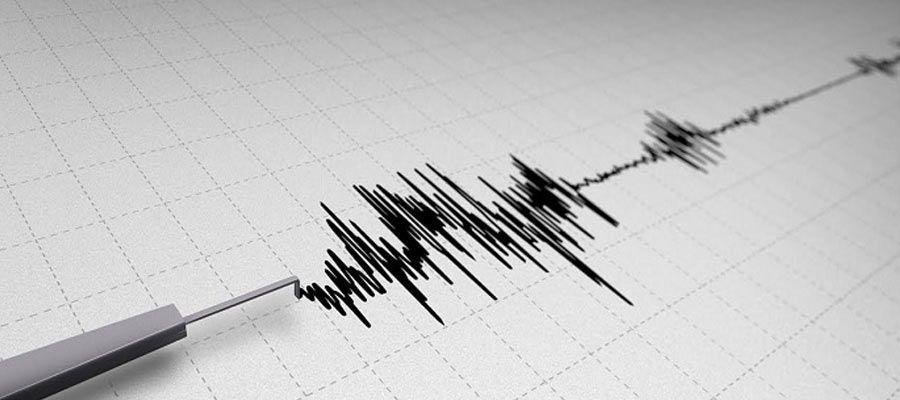# Logarithms Outside School

We learn logarithms every day in school and we think we will never use them in real life unless we become astronauts, but what if we were using logarithms daily without paying attention to how important they are.

### Definitions of Logarithms

A logarithm is the value of a number based on another, for example 10,000 to the base of 10

10000 = 10 x 10 x 10 x 10 so the logarithm of 10000 to the base of 10 is 4.

10000 = 100 x 100 so the logarithm of 10000 to the base of 100 is 2.

### Using logarithms

Logarithms were invented in the 16th century to simplify complicated mathematical problems and calculations, but despite the very advanced computers that we have today, logarithms are still used in a wide range of applications. Here are some uses of logarithms today:

### Richter Magnitude Scale:This is a logarithmic scale with the base of 10, which means that an earthquake with a magnitude of 5 on the Richter’s scale is stronger than the 4-magnitude earthquake by 10 times, and stronger than the 3-magnitude earthquake by 100 and so on.

The harshest earthquake ever recorded had a magnitude of 9.5, and the earthquake caused by the asteroid that hit the planet and caused the extinction of dinosaurs is estimated to have had a magnitude of 13 which is 1,000,000 stronger than the average earthquakes today.

### Simplify Counting• The logarithm of 100 to the base of 10 is 2
• The logarithm of 1000 to the base of 10 is 3
• The logarithm of 1000000 to the base of 10 is 6
• The logarithm of “The number of atoms in the universe” to the base of 10 is 80

### pH Scale (acidity scale)Most teachers do not explain the famous 1-14 pH scale and that it is a logarithmic based scale, for example pH2 is 10 times more acidic than pH1 and pH3 is 100 times more acidic than pH1.

### Graphs and MapsLogarithms are a great tool for scaling when working with graphs and maps, where it helps analyze them on the small paper scale and compare areas with different data and information.

Do you still think logarithms are only used in schools?